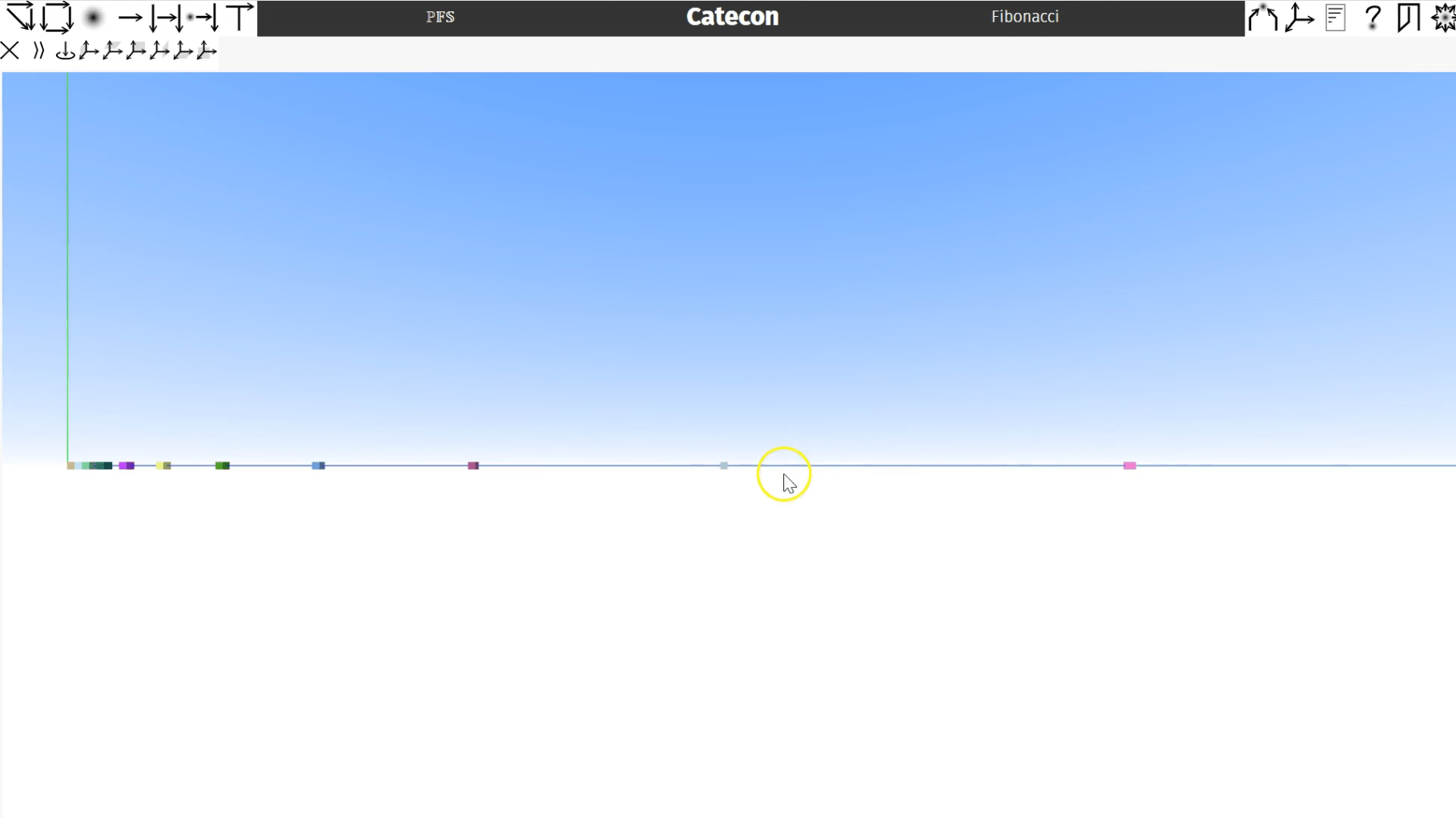# Catecon: Fibonacci

Let’s try to see the Fibonacci numbers.

As you know, the Fibonacci number of n is the Fibonacci number of n-1 plus the Fibonacci number of n-2.

Looks like a natural use of recursion so buckle up.

Let’s start by having our own Fibonacci diagram.

Drag the natural numbers onto the diagram.

Control-drag to form an identity morphism and edit it.

Set 0 to map to 1 and 1 to map to 1 for the Fibonacci numbers of 0 and 1.

Now rename the morphism to Fibonacci.

Control-drag off two copies of it that we’ll need for the recursion.

Select the domain and see where we can go.

Select the predecessor.

Now select that codomain, see where we can go, and select the diagonal to get two copies of n-1.

Control-drag a copy of the predecessor and hover over Fibonacci until the predecessor turns blue.

Drop it to the form the product.

Select the domain of the product and fuse it to the delta’s codomain.

Control-drag from an N to form an identity and detach it.

Drag it over the other Fibonacci morphism and drop it to form a product of the two.

Fuse its domain to the other product’s codomain.

Now choose the last product’s codomain and see where we can go from there.

Addition is a good choice and fuse its codomain with the original Fibonacci morphism.

Select the first predecessor and shift-click the following morphisms and form the composite in the same hom-set as Fibonacci.

Now select Fibonacci and shift-click the composite.

We see the recursion button and click it.

Perhaps we should test this.

Control-drag from an N to from an identity, detach it, and fuse the codomain to Fibonacci’s domain.

Edit the morphism and set a range from 0 to 10 starting at 0.

Select Fibonacci’s codomain, see where we can go, and select conversion to string.

Then from there, we go to tty.

Select these morphisms in order and create the composite.

Evaluate the composite.  That didn’t take long.  We see on the tty some numbers that look somewhat Fibonacci.

We see 2 is the sum of 1 plus 1, and 3 is the sum of 2 plus 1, and so on.

We got to one hundred factorial.  Can we get to the one-hundredth Fibonacci number?  Not so easily.

Check it out.  Clear our test data and map 0 to 30.

Rerun the test morphism.  That took a bit longer.

Now clear the test data again and try a value of 34 and evaluate.

That took a lot longer.

Since each invocation of the Fibonacci morphisms itself invokes two Fibonacci morphisms, the number of morphisms is growing exponentially, and that hurts.

Perhaps we should visualize these numbers to see that they have the way of the Fibonacci’s.

To start let’s set our test data to go from 0 to 12.

Select Fibonacci’s codomain again and go from there.

We see a morphism from N to 3D.  Select it.

Now form the composite of the test data, Fibonacci, and the morphism to 3D.

Evaluate it and expand the screen.

Along the blue axis the Fibonacci values are shown as a cube of a random color.

Though this is not an isometric view, we can get some idea of the correctness of the generated numbers.

As you see, the distance between these two cubes is about the sum of the distances of the two prior segments.

Then the next segment appears to be the sum of the prior two.

Are they gone?

Let’s play in 3D.

Get some nats.

Make a new object of three nats.

Drag the nat to the product of the three.

Edit the data.

Add a random range from zero to 999.

Give zero for all mins and one hundred for all maxs.  Now we have a bunch of random triplets.

From the product, go to 3D.

Form the composite and evaluate.